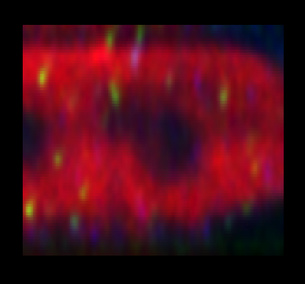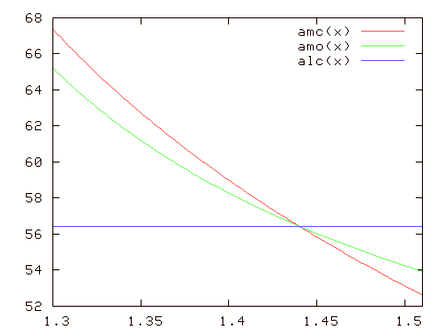## Geometrical distortion: the Fishtank Effect

A geometrical distortion happens whenever distances in the sample under our microscope as we record them are actually different in reality.

This happens for example in the presence of a Refractive Index mismatch (which also provokes Spherical Aberration!!!). The objective moves along the optical axis a certain distance (a z step that is recorded in the data file), but the focus shifts inside the sample at an actual different step. Therefore objects will appear shortened (as in a fish tank when the objects in the water are viewed from the air) or elongated in the microscope, depending on the ratios of the indices.

In most cases the z-sampling as specified in the raw datafile is the nominal sampling distance, i.e. the distance the table or objective actually moved in Z without taking foreshortening due to Refractive Index Mismatch into account. The Huygens Software will automatically adapt the PSF to this situation, but it will not modify the image geometry (see Focal Shift Correction).

Assuming that you have an oil lens (RI=1.51) and a watery specimen (RI=1.31) then the object will appear longer. On the other hand, the change from air (RI = 1) to water (RI = 1.31) (for example the straw in your glass of lemonade) will give a shortened, smaller object (= the fishtank effect).

An example, if you have a Z sampling of 180 nm and a water lens with an air medium the correct z-sampling with correction for geometrical distorsion is (1.31/1.00 = 1.31= the elongation factor) so the corrected dimensions for the geometrical dimensions are then 180 divided by 1.31 = 137 nm.
See for another example the two images just below.

So generally speaking, After deconvolution the remaining geometric distortion can be corrected by multiplying the (nominal) z-Sampling Distance by the ratio of the objective and immersion refractive indices. If the objective has a higher RI then the medium (thus elongating the object) you will get a correction factor < 1, if the objective has a lower RI then the medium (thus shortening the object) you will get a correction factor > 1.

To get this geometrical correction factor taking effect you then simply change the Z dimension in the meta data overview into the newly calculated one. Look at the geometrically corrected image again in one of the viewers to see the geometrical distorsion corrected.Lateral (XZ) views of a 3D confocal image deconvolved with Huygens. The first (left) image is still elongated in a factor of ~ 1.13 along the optical axis due to a refractive index mismatch between the oil lens and the water medium (1.51 / 1.33). The second (right) image: after correcting the distortion by dividing the Z sampling by 1.13 (the elongation factor). So assuming that the nominal z-sampling is 300 nm the corrected for geomatric distortion z-sampling = 265 nm (calculation: 300 nm divided by (1.33/1.51) = 265 nm)

See Focal Shift Correction.

### What happens to the aperture angle?

If distances change, then also the aperture angle (the angle subtended by the lens at the focus, but not the Numerical Aperture) will change!!! It is like the apparent bending of a stick half-inmmersed in water...Image from plus magazine

Let's consider a set up with Numerical Aperture NA = 1.2 and Lens Immersion Medium with Refractive Index nl = 1.44. In the graph below, the x axis is the refractive index nm of the Specimen Embedding Medium, that is mismatched except in the point 1.44.

The red curve is the half-aperture angle (degrees) as it happens in the medium: amc = arcsin(NA/nm). The green curve is what is actually observed, in a Paraxial Simplification, due to the geometry distortion caused by the refractive mismatch: amo = atan( (nm/nl) * tanx ). The blue line is a reference: the angle that should be observed in a total match situation: alc = arcsin(NA/nl).When the sample medium is "denser", we are in the case of the fish tank effect: objects are collapsed on the axial direction, thus angles look wider. The green curve goes above the red one.

When it is the other way around objects look elongated, and the apparent angles are narrower.

This effect is much enhanced it the real case where not only paraxial rays are taken into account. Then the apparent angle (green curve), on the left side of the graph, can even go below the blue line!!!